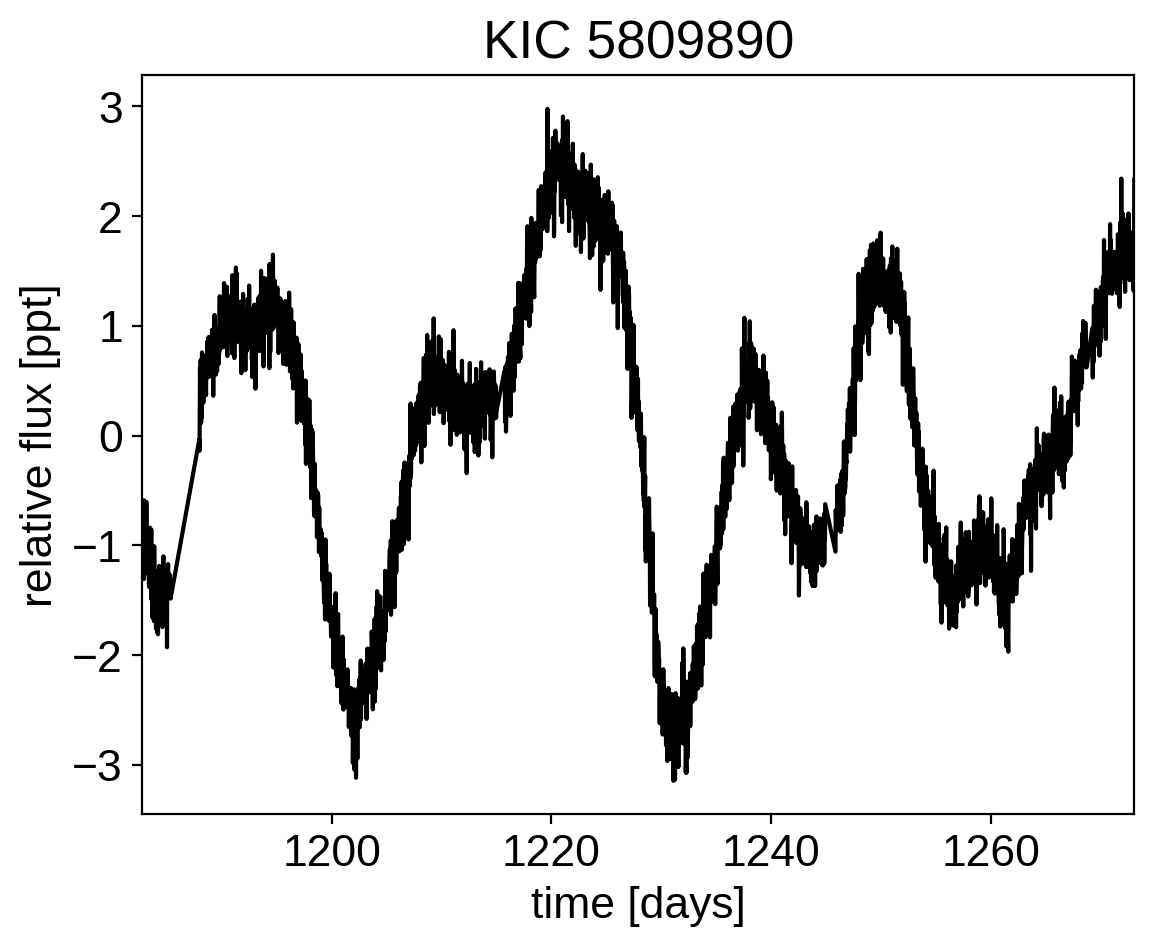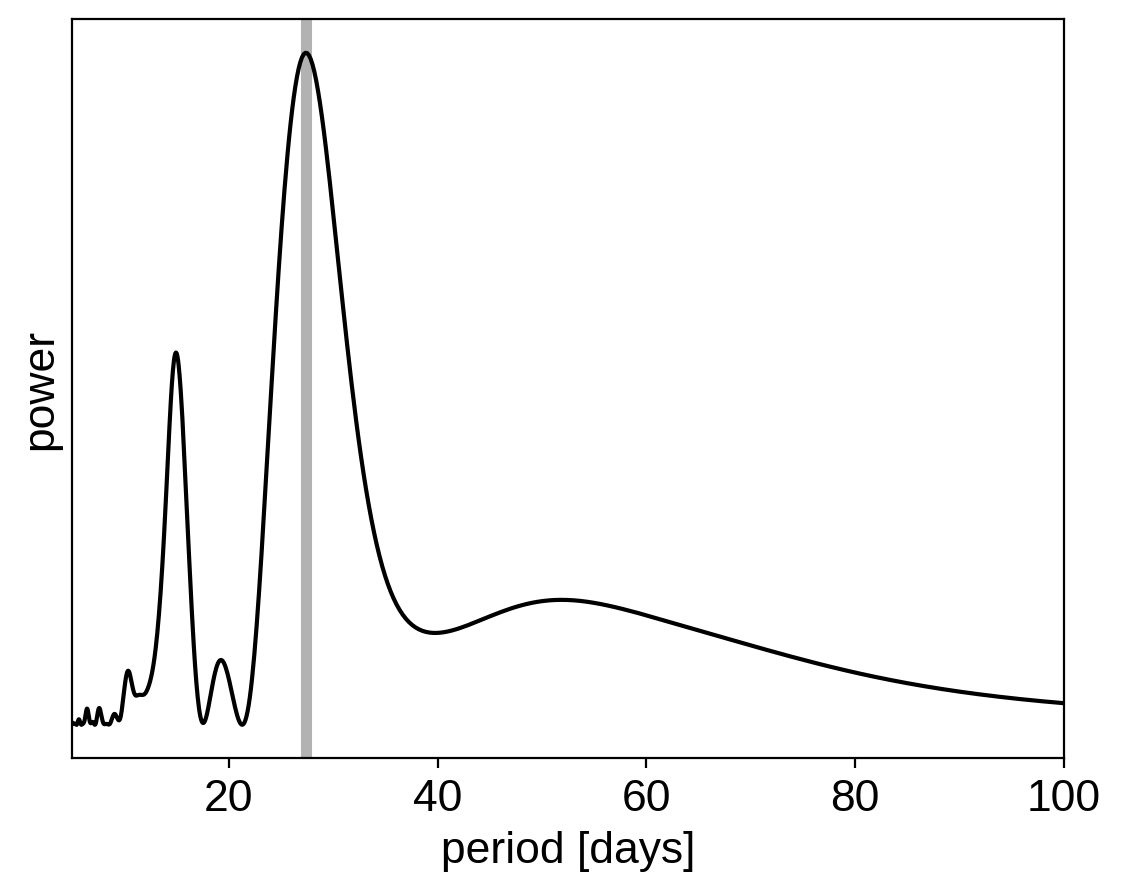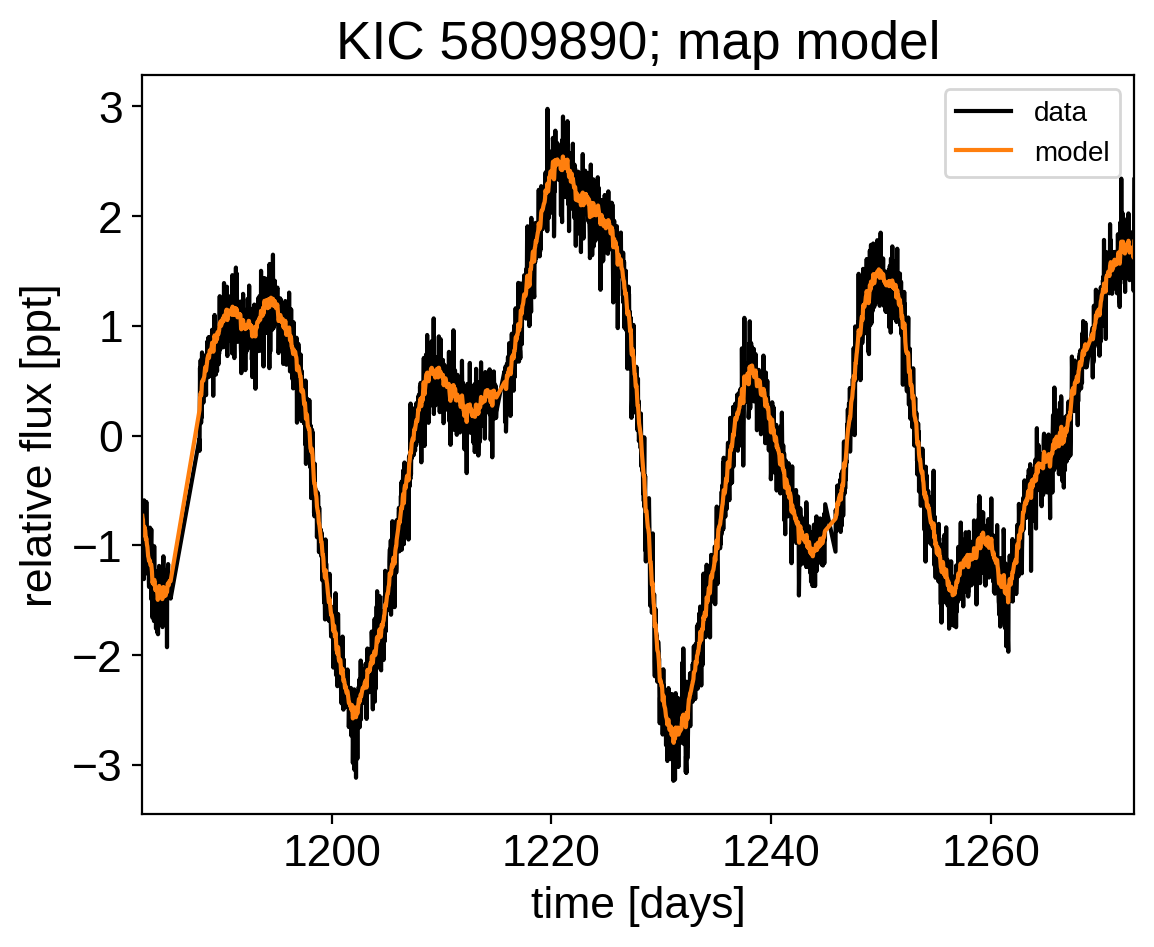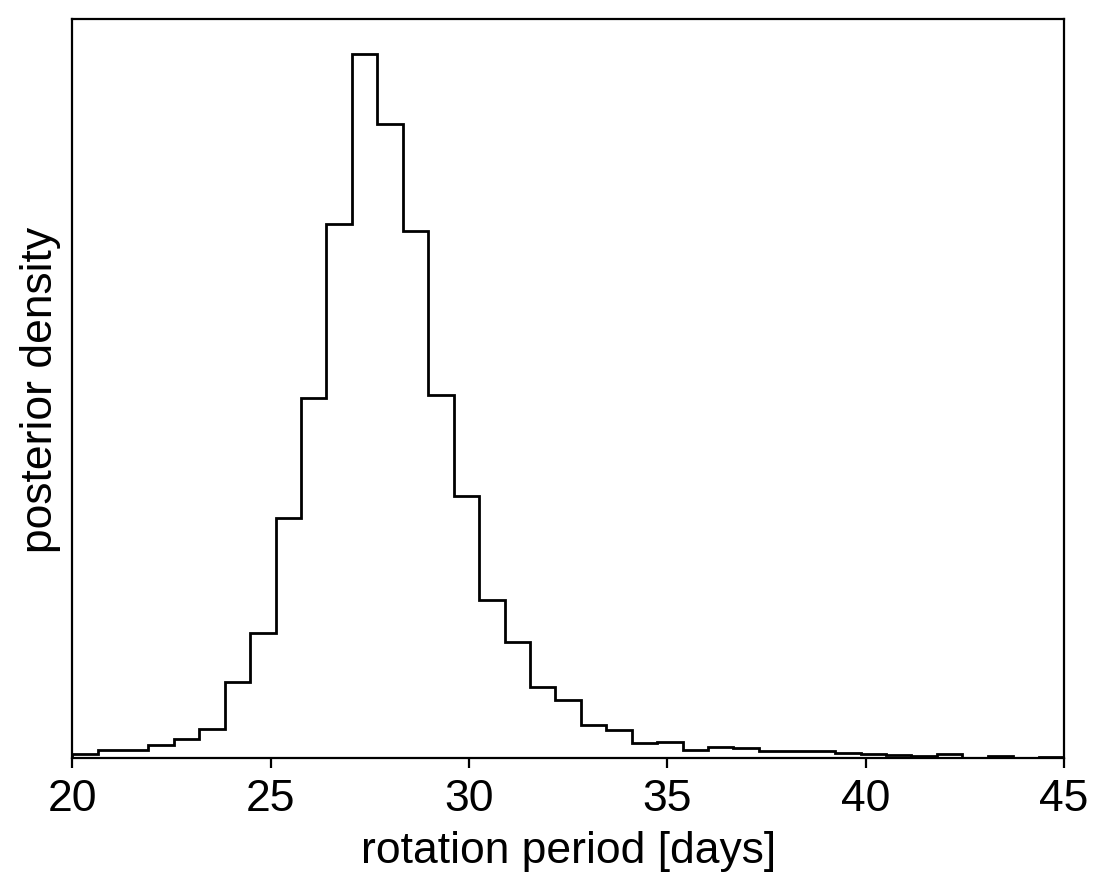Note

This tutorial was generated from an IPython notebook that can be downloaded here.

# Gaussian process models for stellar variability¶

When fitting exoplanets, we also need to fit for the stellar variability and Gaussian Processes (GPs) are often a good descriptive model for this variation. PyMC3 has support for all sorts of general GP models, but exoplanet includes support for scalable 1D GPs (see Scalable Gaussian processes in PyMC3 for more info) that can work with large datasets. In this tutorial, we go through the process of modeling the light curve of a rotating star observed by Kepler using exoplanet.

import numpy as np
import lightkurve as lk
import matplotlib.pyplot as plt

)
lc = lcf.PDCSAP_FLUX.normalize().remove_nans().remove_outliers()

x = np.ascontiguousarray(lc.time, dtype=np.float64)
y = np.ascontiguousarray(lc.flux, dtype=np.float64)
yerr = np.ascontiguousarray(lc.flux_err, dtype=np.float64)
mu = np.mean(y)
y = (y / mu - 1) * 1e3
yerr = yerr * 1e3 / mu

plt.plot(x, y, "k")
plt.xlim(x.min(), x.max())
plt.xlabel("time [days]")
plt.ylabel("relative flux [ppt]")
plt.title("KIC 5809890");## A Gaussian process model for stellar variability¶

This looks like the light curve of a rotating star, and it has been shown that it is possible to model this variability by using a quasiperiodic Gaussian process. To start with, let’s get an estimate of the rotation period using the Lomb-Scargle periodogram:

import exoplanet as xo

results = xo.estimators.lomb_scargle_estimator(
x, y, max_peaks=1, min_period=5.0, max_period=100.0, samples_per_peak=50
)

peak = results["peaks"]
freq, power = results["periodogram"]
plt.plot(1 / freq, power, "k")
plt.axvline(peak["period"], color="k", lw=4, alpha=0.3)
plt.xlim((1 / freq).min(), (1 / freq).max())
plt.yticks([])
plt.xlabel("period [days]")
plt.ylabel("power");Now, using this initialization, we can set up the GP model in exoplanet. We’ll use the exoplanet.gp.terms.RotationTerm kernel that is a mixture of two simple harmonic oscillators with periods separated by a factor of two. As you can see from the periodogram above, this might be a good model for this light curve and I’ve found that it works well in many cases.

import pymc3 as pm
import theano.tensor as tt

with pm.Model() as model:

# The mean flux of the time series
mean = pm.Normal("mean", mu=0.0, sd=10.0)

# A jitter term describing excess white noise
logs2 = pm.Normal("logs2", mu=2 * np.log(np.mean(yerr)), sd=2.0)

# A term to describe the non-periodic variability
logSw4 = pm.Normal("logSw4", mu=np.log(np.var(y)), sd=5.0)
logw0 = pm.Normal("logw0", mu=np.log(2 * np.pi / 10), sd=5.0)

# The parameters of the RotationTerm kernel
logamp = pm.Normal("logamp", mu=np.log(np.var(y)), sd=5.0)
BoundedNormal = pm.Bound(pm.Normal, lower=0.0, upper=np.log(50))
logperiod = BoundedNormal("logperiod", mu=np.log(peak["period"]), sd=5.0)
logQ0 = pm.Normal("logQ0", mu=1.0, sd=10.0)
logdeltaQ = pm.Normal("logdeltaQ", mu=2.0, sd=10.0)
mix = xo.distributions.UnitUniform("mix")

# Track the period as a deterministic
period = pm.Deterministic("period", tt.exp(logperiod))

# Set up the Gaussian Process model
kernel = xo.gp.terms.SHOTerm(log_Sw4=logSw4, log_w0=logw0, Q=1 / np.sqrt(2))
kernel += xo.gp.terms.RotationTerm(
log_amp=logamp, period=period, log_Q0=logQ0, log_deltaQ=logdeltaQ, mix=mix
)
gp = xo.gp.GP(kernel, x, yerr ** 2 + tt.exp(logs2))

# Compute the Gaussian Process likelihood and add it into the
# the PyMC3 model as a "potential"
pm.Potential("loglike", gp.log_likelihood(y - mean))

# Compute the mean model prediction for plotting purposes
pm.Deterministic("pred", gp.predict())

# Optimize to find the maximum a posteriori parameters
map_soln = xo.optimize(start=model.test_point)

optimizing logp for variables: [mix, logdeltaQ, logQ0, logperiod, logamp, logw0, logSw4, logs2, mean]
163it [00:12, 13.35it/s, logp=7.031724e+02]
message: Desired error not necessarily achieved due to precision loss.
logp: 267.25686032200514 -> 703.1723809089232


Now that we have the model set up, let’s plot the maximum a posteriori model prediction.

plt.plot(x, y, "k", label="data")
plt.plot(x, map_soln["pred"], color="C1", label="model")
plt.xlim(x.min(), x.max())
plt.legend(fontsize=10)
plt.xlabel("time [days]")
plt.ylabel("relative flux [ppt]")
plt.title("KIC 5809890; map model");That looks pretty good! Now let’s sample from the posterior using exoplanet.get_dense_nuts_step().

np.random.seed(42)
with model:
trace = pm.sample(
tune=2000,
draws=2000,
start=map_soln,
step=xo.get_dense_nuts_step(target_accept=0.9),
)

Multiprocess sampling (4 chains in 4 jobs)
NUTS: [mix, logdeltaQ, logQ0, logperiod, logamp, logw0, logSw4, logs2, mean]
Sampling 4 chains: 100%|██████████| 16000/16000 [06:45<00:00, 39.49draws/s]
There were 2 divergences after tuning. Increase target_accept or reparameterize.
There were 2 divergences after tuning. Increase target_accept or reparameterize.
There were 5 divergences after tuning. Increase target_accept or reparameterize.
The number of effective samples is smaller than 25% for some parameters.


Now we can do the usual convergence checks:

pm.summary(
trace,
varnames=[
"mix",
"logdeltaQ",
"logQ0",
"logperiod",
"logamp",
"logSw4",
"logw0",
"logs2",
"mean",
],
)

mean sd mc_error hpd_2.5 hpd_97.5 n_eff Rhat
mix 0.638907 0.246249 0.005999 0.178848 0.999876 1388.904252 1.000946
logdeltaQ 0.622804 9.218069 0.181064 -17.817309 19.667857 2368.845797 1.000144
logQ0 0.781421 0.536186 0.007963 -0.247969 1.821605 3670.677635 0.999928
logperiod 3.326002 0.096711 0.002497 3.145160 3.528834 1366.843586 1.000468
logamp 0.384757 0.606889 0.013657 -0.588614 1.632578 1373.325178 1.000085
logSw4 6.610959 0.747819 0.012153 5.128654 8.023461 3602.498378 1.000397
logw0 3.861721 0.195403 0.002990 3.478037 4.236481 4046.885861 1.000415
logs2 -6.207327 0.682157 0.014721 -7.640965 -5.205967 2293.592072 1.000621
mean -0.014214 0.202787 0.002961 -0.418842 0.384741 4635.502757 0.999902

And plot the posterior distribution over rotation period:

period_samples = trace["period"]
bins = np.linspace(20, 45, 40)
plt.hist(period_samples, bins, histtype="step", color="k")
plt.yticks([])
plt.xlim(bins.min(), bins.max())
plt.xlabel("rotation period [days]")
plt.ylabel("posterior density");